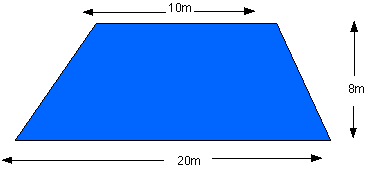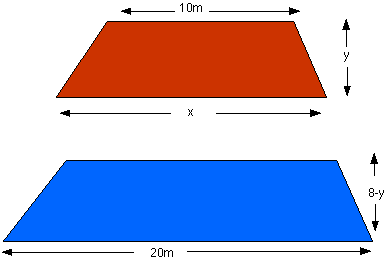#### You may also like### At a Glance

The area of a regular pentagon looks about twice as a big as the pentangle star drawn within it. Is it?### Six Discs

Six circular discs are packed in different-shaped boxes so that the discs touch their neighbours and the sides of the box. Can you put the boxes in order according to the areas of their bases?### Equilateral Areas

ABC and DEF are equilateral triangles of side 3 and 4 respectively. Construct an equilateral triangle whose area is the sum of the area of ABC and DEF.

# Dividing the Field

##### Age 14 to 16Challenge Level

A trapezium has area equal to half the sum of the lengths of the parallel edges times the perpendicular distance between them. The original field has area $h(a+b)/2$ and we are looking for a field with area $h(a+b)/4$.

One solution would be to join the midpoints of the parallel edges with a straight line.

There are lots of other solutions. The farmer might want to start from a gate on the edge of length $a$ , at a distance $d$ from one corner, and $(a-d)$ from the other. The dividing line would have to be drawn to a point on the opposite side of the field at a distance $(a+b)/2$ from the corner.

Some people tried to solve a harder problem, that is to split the field by a line parallel to the parallel edges of the field. A particular solution to this was sent in by Jack , Henry, Paul and Matthew from Smithdon High School. It is shown below.Area of original field $= A = 8 \times(10 + 20) / 2 = 120$m$^2$

Therefore area of each new field $= 60$ m$^2$

Let the height' of the first field be $y$ metres and the bottom edge be $x$ metres.

This means that the height' of the second field must be $(8 - y)$ and the top edge must be $x$ metres.For the first field:

$60=y \times (10-x )/2$- (1)

and for the second field:

$60 = (8-y) \times(20+x)/2$ - (2)

Equation (1) can be rearranged to give:

y = 120 / (10+ x ) - (3)

and equation (2) gives:

120 = (8 - y ) (20 + x ) - (4)

Combining equations (3) and (4) gives:

$120 = (8-120/[10+x])(20+x)$ - (5)

so

$120(10+x)=(8[10+x]-120)(20+x)$

and

$1200+120x=(80+8x-120)(20+x)$

which leads to

$150+15x=(x-5)(x+20)$

and $150+15x=x^2+15x-100)$

so $x^2 = 250$m$^2$

which gives $x=15.8$m (3 s.f.)

and in equation (3) $y=120/(10+x)$ so $y= 4.65$m (3 s.f.)

A further challenge: The solution $x^2 = (a^2 + b^2)/2$ is very special mathematically because it is the same whatever the value of the distance $h$. The language mathematicians use is to say that "the solution is independent of $h$''. Can you explain why this happens in this particular problem? If you want a hint then you could use the fact that when you enlarge a shape with a linear scale factor $s$ then the area is enlarged by a scale factor $s^2$. Look out for more about this problem in a future article on areas and scaling.

Correct solutions to the problem were received from Nicholas - South Greenhoe Middle School ;Heacham Middle School; Lucy and Sarah - Archbishop Sancroft High School